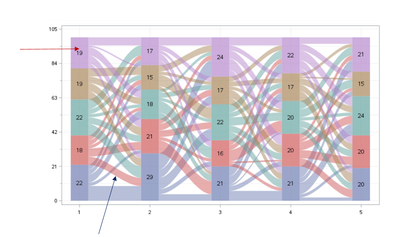## multiple labels in sgplot (polygon)

Dear all,

assuming I had this code which plots a sankey chart

``````proc sgplot data=myplotdata noborder;
polygon x=x y=y id=id / fill nooutline group=group transparency=0.3 name='p' label=count labelloc=insidebbox ;
xaxistable nodes / x=x_label location=inside position=top nolabel title="Sankey Chart" valueattrs=(size=12pt) titleattrs=(size=12pt) ;
xaxis min=0 max=%sysevalf(100+5) values=(0 to 100 by 10) display=none valueshint;
yaxis min=0 max=100 reverse values=(0 to 100 by 10) display=none;
keylegend 'p'/ title=" " noborder location=outside position=bottom exclude=('') valueattrs=(size=12pt) titleattrs=(size=12pt);
run;``````

I  am able to label the bar segments with label=count. I will also like to label the nodes leaving the segments (variable=n_move). My question is, is it possible to label using multiple variables (in this case count and n_move) in sgplot?

if yes, how ?The red arrow indicates label=count  and the blue arrow indicates label=n_move showing the number of patients leaving that group

2 REPLIES 2

## Re: multiple labels in sgplot (polygon)

If you have x, y coordinates associated where you want the label placed a TEXT plot would work.

Or provide data so we can run your code and see what might be possible. Sometimes these extra label steps are easy, sometimes you need to add variables or rearrange data a bit.

You can try duplicating your plot statement with a the label changed to the other variable. If that obscures your plot too much try turning the transparency up in BOTH ploygon statements as the overlaps will get more saturation.

I hope you are making this a relatively large graphic as you are going to have a lot of text floating around, likely with collisions. Are you sure that you want those skinny connections with text?

## Re: multiple labels in sgplot (polygon)

@ballardw Thankyou, I will try that

Discussion stats
• 2 replies
• 133 views
• 0 likes
• 2 in conversation